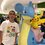# Generating Function for Partitions

When I was at the ARML Power Contest, I came upon question 11 which stated: Explain why the number of partitions of $n$ into all different parts is given by the coefficient of $x^{n}$ in the expansion of the product $\left(1+x\right)\left(1+x^{2}\right)\left(1+x^{3}\right)...\left(1+x^{n}\right)$.

The answer explains how, when the product is expanded but like terms are not collected, each different term that is $x^{n}$ is formed from adding the powers of several terms from the initial semi-factored form.

I was wondering, does anybody know if there's a generating function or regular old function for the total number of partitions a number can be split into? I tried finding one in terms of sigma, then started working on a Python solution, but it doesn't seem like there is. The formulas I used had to do with combinations and even the same kind of logic that is used to determine the number of ways a certain number can be rolled using any number of dice. If an experienced computer scientist can come up with a great Python or Java version, that would be great also.

Thanks,

Tristan ShinNote by Tristan Shin
7 years, 5 months ago

This discussion board is a place to discuss our Daily Challenges and the math and science related to those challenges. Explanations are more than just a solution — they should explain the steps and thinking strategies that you used to obtain the solution. Comments should further the discussion of math and science.

When posting on Brilliant:

• Use the emojis to react to an explanation, whether you're congratulating a job well done , or just really confused .
• Ask specific questions about the challenge or the steps in somebody's explanation. Well-posed questions can add a lot to the discussion, but posting "I don't understand!" doesn't help anyone.
• Try to contribute something new to the discussion, whether it is an extension, generalization or other idea related to the challenge.
• Stay on topic — we're all here to learn more about math and science, not to hear about your favorite get-rich-quick scheme or current world events.

MarkdownAppears as
*italics* or _italics_ italics
**bold** or __bold__ bold
- bulleted- list
• bulleted
• list
1. numbered2. list
1. numbered
2. list
Note: you must add a full line of space before and after lists for them to show up correctly
paragraph 1paragraph 2

paragraph 1

paragraph 2

[example link](https://brilliant.org)example link
> This is a quote
This is a quote
    # I indented these lines
# 4 spaces, and now they show
# up as a code block.

print "hello world"
# I indented these lines
# 4 spaces, and now they show
# up as a code block.

print "hello world"
MathAppears as
Remember to wrap math in $$ ... $$ or $ ... $ to ensure proper formatting.
2 \times 3 $2 \times 3$
2^{34} $2^{34}$
a_{i-1} $a_{i-1}$
\frac{2}{3} $\frac{2}{3}$
\sqrt{2} $\sqrt{2}$
\sum_{i=1}^3 $\sum_{i=1}^3$
\sin \theta $\sin \theta$
\boxed{123} $\boxed{123}$

## Comments

Sort by:

Top Newest

For regular partitions, the generating function is $\frac{1}{(1 - x)(1 - x^2)(1 - x^3) \dotsm}.$ See http://en.wikipedia.org/wiki/Partition%28numbertheory%29

- 7 years, 5 months ago

Log in to reply

Thanks! It seems that just a little bit of manipulation can cause wonderful generating functions!

- 7 years, 5 months ago

Log in to reply

×

Problem Loading...

Note Loading...

Set Loading...